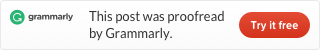Categories

# LeetCode 56. Merge Intervals [sort] Java

Given a collection of intervals, merge all overlapping intervals.

Example 1:

```Input: [[1,3],[2,6],[8,10],[15,18]]
Output: [[1,6],[8,10],[15,18]]
Explanation: Since intervals [1,3] and [2,6] overlaps, merge them into [1,6].```

Example 2:

```Input: [[1,4],[4,5]]
Output: [[1,5]]
Explanation: Intervals [1,4] and [4,5] are considered overlapping.```

NOTE: input types have been changed on April 15, 2019. Please reset to default code definition to get new method signature.

Look at example 1, I draw this picture:

We can find out if we sort the intervals by the left number, we can easily solve this problem. In this situation, when the right number of one interval is smaller or equal the left number of next interval, we know them are overlapping. So we get function isOverlap:

And it is easy to define a merge function to merge two overlapping intervals:

Then we can write the main merge function, use Arrays.sort to sort intervals, we only need to implement a compare method, only need to compare the left number of intervals.

Then we loop every interval. First, make the first interval as current, when start loop from the second interval. If the current interval is overlapping the loop interval, merge them; if not, we put the current interval to the result array and make the loop interval as current;

Then output the ArrayList as an array.